## Monday, January 26, 2015

### T-SQL Last Non Empty

SQL Server 2012 | SQL Server 2014 | PDW/APS 2012

Recently we needed to calculate something like a ‘last non empty’ value in a T-SQL query. This blog post is about the solution we ended up with as an alternative to the classic pattern involving sub-queries.

To illustrate the task let’s first look at some data: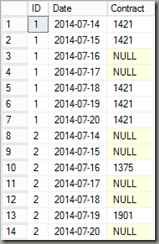The extract shows a contract table with some gaps. The task is to fill the gaps with the last non empty contract of the same id. So, here is the final result: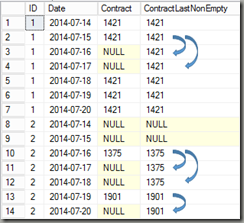As you can see, apart from the first days for id 2 which don’t have a last value, all gaps have been filled.

In order to fill the gaps using T-SQL window functions, the idea is to calculate the number of steps we need to go back for each null value to catch the corresponding last value. In the following screenshot, I’m showing this value as the last column: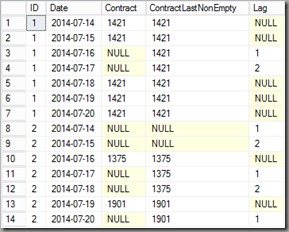For example, for ID 1, Date 2014-07-17 we have to go two rows back (2014-07-15) to get the last non empty value. For the first two dates for ID 2 we also have a lag-value, however there is no corresponding row. Looking at the lag columns it somewhat looks like a row_number over rows with a contract value of NULL. Actually, looking at ID 2 there may be more than one gap (NULL values) so it’s more like a row number over groups of contracts. To determine those groups we need to find changes in the contract over time. So let’s start with this first.

with
C1 as
(select ID, Date, Contract
, iif(isnull(Contract,'') <> isnull(lag(Contract,1) over (partition by ID order by Date),''),1,0) ContractChange
from  [dbo].[Contracts])

select * from C1 order by ID, Date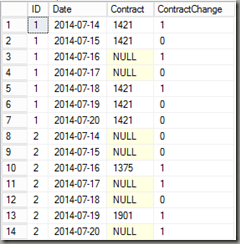Using the lag window-function I added a new column ‘ContractChange’ that gives 1 whenever the contract changes and 0 otherwise. The next step is to calculate a running total of the column to build up groups of contracts:

with
C1 as…
C2 as
(select ID, Date, Contract, ContractChange,
sum(ContractChange) over (partition by id order by Date) ContractGroup
from C1)

select * from C2 order by ID, Date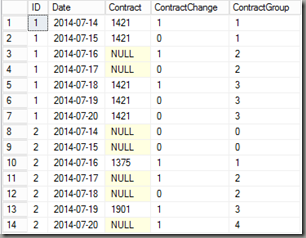The new column ‘ContractGroup’ now calculates a value that increments whenever the contract changes. We can now calculate a row_number using the ContractGroup column as the partition:

with
C1 as…
C2 as…
C3 as
(select ID, Date, Contract, ContractChange, ContractGroup,
row_number() over (partition by id, ContractGroup order by Date) LastContractLag
from C2)

select * from C3 order by ID, Date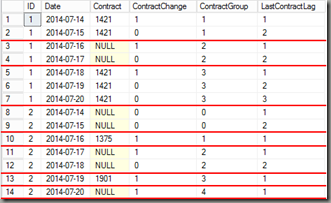And actually, the LastContractLag column here is already the value we need for the lag-function to get to the non-empty value. So here is the final query (including the intermediate calculations from above):

with
C1 as
(select ID, Date, Contract
, iif(isnull(Contract,'') <> isnull(lag(Contract,1) over (partition by ID order by Date),''),1,0) ContractChange
from  [dbo].[Contracts])
,
C2 as
(select ID, Date, Contract, ContractChange,
sum(ContractChange) over (partition by id order by Date) ContractGroup
from C1)
,
C3 as
(select ID, Date, Contract, ContractChange, ContractGroup,
row_number() over (partition by id, ContractGroup order by Date) LastContractLag
from C2)

select ID, Date, Contract
,iif(Contract is null, lag(Contract,LastContractLag) over (partition by id order by Date),Contract) ContractLastNonEmpty
from C3
order by ID, Date

The output of this query is shown above (final result). And again this is a good example of the power of window functions.

Conclusion

In our situation, this solution performed much better than a sub-query approach, but depending on the table layout and the amount of data, other approaches may still be better, so you may want to try different patterns for solving this problem.